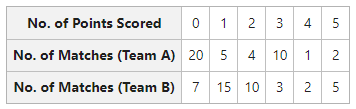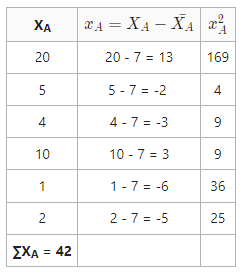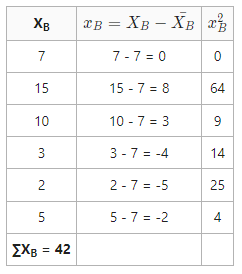GFG App
Open AppBrowser
Continue

# Coefficient of Variation: Meaning, Formula and Examples

### What is Coefficient of Variation?

As Standard Deviation is an absolute measure of dispersion, one cannot use it for comparing the variability of two or more series when they are expressed in different units. Therefore, in order to compare the variability of two or more series with different units it is essential to determine the relative measure of Standard Deviation. Two of the relative measures of Standard Deviation are Coefficient of Standard Deviation and Coefficient of Variation.

Coefficient of Variation is a relative measure introduced by Karl Pearson (also known as Karl Pearson’s Coefficient of Variation) through which two or more groups of similar data are compared with respect to stability or homogeneity or consistency. It is the most appropriate measure and indicates the relationship between the standard deviation and the arithmetic mean of the given distributions/series. Coefficient of Variation is expressed in terms of percentage and can be determined using the following formula:Where,

C.V. = Coefficient of Variation

σ = Standard Deviation= Arithmetic Mean

In simple terms, the Coefficient of Variation is 100 times of Coefficient of Standard Deviation. The distribution/series for which the coefficient of variation is greater is more variable (less homogeneous, less consistent, less stable, or less uniform).

#### Example 1:

The number of points scored by two teams in a hockey match is given below. With the help of Coefficient of Variation, determine which team is more consistent.#### Solution:

Coefficient of Variation (Team A):Coefficient of Variation (Team A) =C.V. (Team A) = 92.5%

Coefficient of Variation (Team B):Coefficient of Variation (Team B) =C.V. (Team B) = 62.7%

Coefficient of Team B is less, i.e., 62.7%, so Team B is more consistent.

#### Example 2:

Coefficient of Variation and Standard Deviation of two series X and Y are 55.43% and 48.86%, and 25.5 and 24.43, respectively. Find the means of series X and Y.

#### Solution:Mean of Series XMean of Series YMean of Series X = 46, Mean of Series Y = 50

My Personal Notes arrow_drop_up